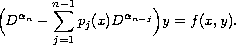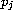Electron. J. Diff. Eqns., Vol. 2006(2006), No. 129, pp. 1-12.

### Existence of positive solutions for multi-term non-autonomous fractional differential equations with polynomial coefficients Azizollah Babakhani, Varsha Daftardar-Gejji

Abstract:
In the present paper we discuss the existence of positive solutions in the case of multi-term non-autonomous fractional differential equations with polynomial coefficients; the constant coefficient case has been studied in . We consider the equationWe state various conditions onand's under which this equation has: positive solutions, a unique solution which is positive, and a unique solution which may not be positive.

Submitted July 27, 2005. Published October 16, 2006.
Math Subject Classifications: 26A33, 34B18.
Key Words: Riemann-Liouville fractional derivatives and integrals; normal cone; semi-ordered Banach space; completely continuous operator; equicontinuous set.

Show me the PDF file (252K), TEX file, and other files for this article.Azizollah Babakhani Department of Mathematics University of Mazanderan, Babol, Iran email: babakhani@nit.ac.ir Varsha Daftardar-Gejji Department of Mathematics, University of Pune Ganeshkhind, Pune - 411007, India email: vsgejji@math.unipune.ernet.in